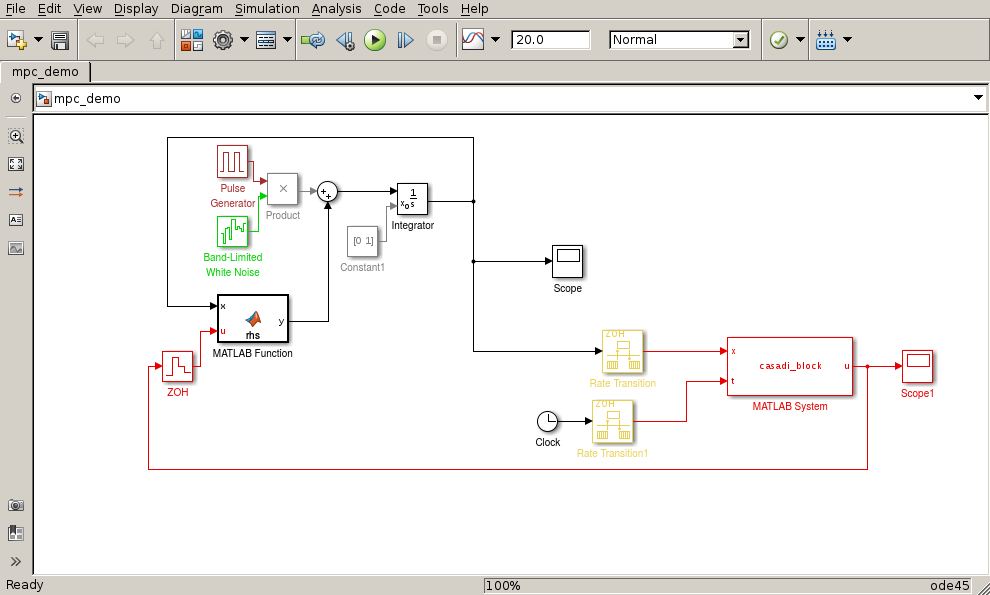CasADi labs is an open-ended platform for sharing knowledge about CasADi. Any user can guest-post by making pull-requests to the blogging repo.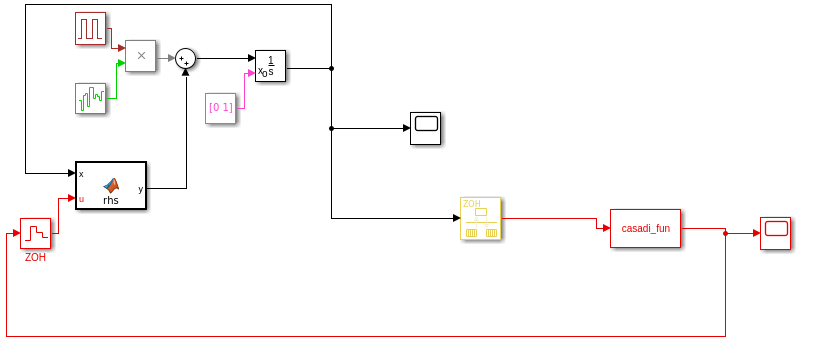In this post, we have a new take on nonlinear MPC in Simulink using CasADi. Interpreter mode In an earlier post on MPC in Simulink, we used an interpreted ‘Matlab system’ block in the simulink diagram. This is flexible, but slow because of interpreter overhead. code-generation mode In an earlier post on S-Functions, we showed how Casadi-generated C code can be embedded efficiently in a Simulink diagram using S-functions. The result is fast, but has restrictions: only SqpMethod combined with Qrqp or Osqp solver can be code-generated (as of 3.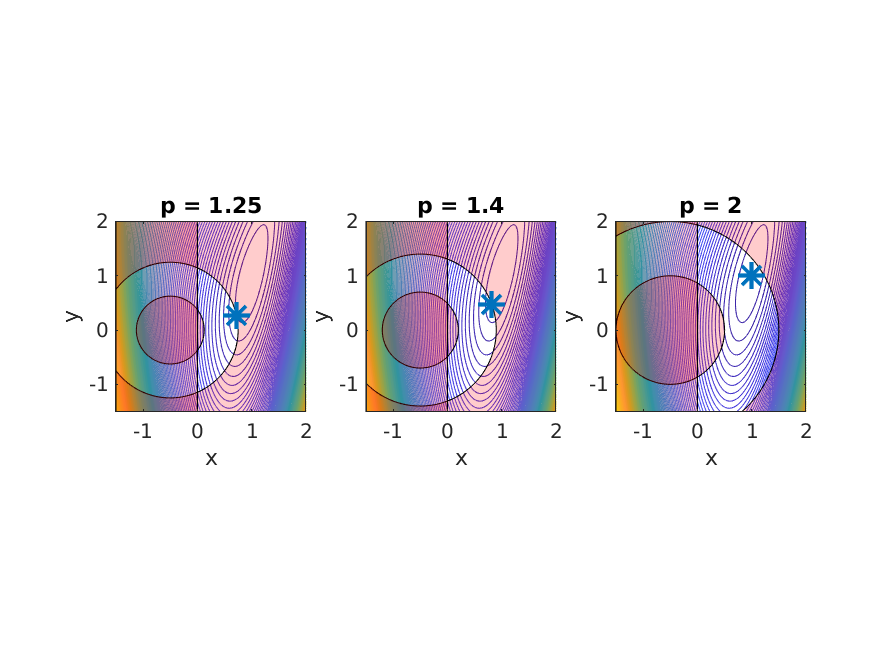##### Sensitivities of parametric NLP
In this post, we explore the parametric sensitivities of a nonlinear program (NLP). While we use ‘Opti stack’ syntax for modeling, differentiability of NLP solvers works all the same without Opti. Parametric nonlinear programming Let’s start by defining an NLP that depends on a parameter $p \in \mathbb{R}$ that should not be optimized for: \begin{align} \displaystyle \underset{x,y} {\text{minimize}}\quad &\displaystyle (1-x)^2+0.2(y-x^2)^2 \newline \text{subject to} \, \quad & \frac{p^2}{4} \leq (x+0.5)^2+y^2 \leq p^2 \newline & x\geq 0 \end{align}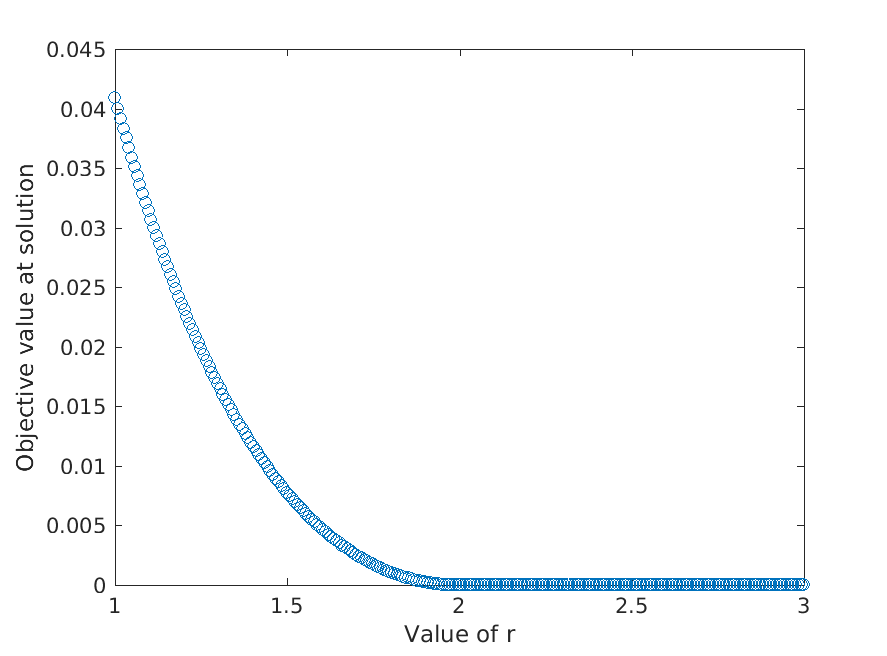##### Parfor
In this post we’ll explore how to use Matlab’s parfor with a CasADi nonlinear program. The nonlinear program (NLP) of interest is the following: \begin{align} \displaystyle \underset{x,y} {\text{minimize}}\quad &\displaystyle (1-x)^2+(y-x^2)^2 \newline \text{subject to} \, \quad & x^2+y^2 \leq r \end{align} Note that $r$ is a free parameter. Our goal is to collect each solution of the NLP as we loop over $r$. We could imagine performing such task when tracing a pareto front of a multi-objective optimization, for which the parameter would be the a weight to combine those objectives.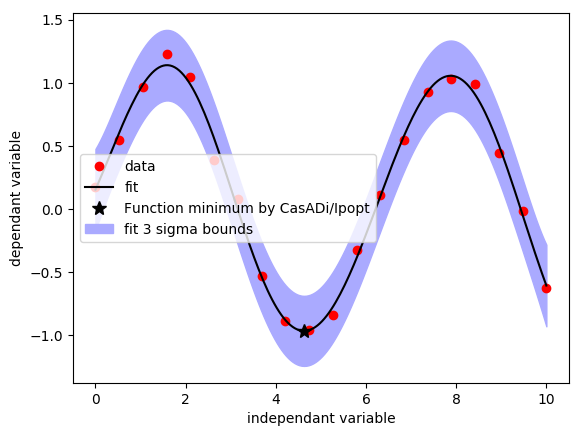In this post we’ll explore how to couple Tensorflow and CasADi. Thanks to Jonas Koch (student @ Applied Mathematics WWU Muenster) for delivering inspiration and example code. One-dimensional regression with GPflow An important part of machine learning is about regression: fitting a (non-)linear model through sparse data. This is an unconstrained optimization problem for which dedicated algorithms and software are readily available. Let’s create some datapoints to fit, a perturbed sine.
##### Breaking free of CasADi's solvers
Once you’ve modeled your optimization problem in CasADi, you don’t have to stick to the solvers we interface. In this post, we briefly demonstrate how we can make CasADi and Matlab’s fmincon cooperate. Trivial unconstrained problem Let’s consider a very simple scalar unconstrained optimization: \begin{align} \displaystyle \underset{x} {\text{minimize}}\quad & \sin(x)^2 \ \end{align} You can solve this with fminunc: fminunc(@(x) sin(x)^2, 0.1) The first argument, an anonymous function, can contain any code, including CasADi code.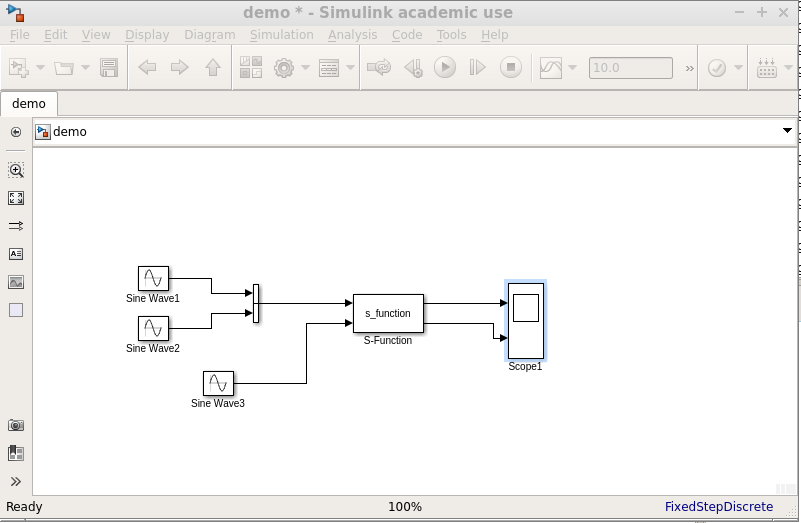While the user guide does explain code-generation in full detail, it is handy to have a demonstration in a real environment like Matlab’s S-functions. The problem We will design a Simulink block that implements a nonlinear mapping from ($\mathbf{R}^2$, $\mathbf{R}$) to ($\mathbf{R}$,$\mathbf{R}^2$): import casadi.* x = MX.sym('x',2); y = MX.sym('y'); w = dot(x,y*x); z = sin(x)+y+w; f = Function('f',{x,y},{w,z}); Code-generating You may generate code from this with: f.generate('f.c') However, we’ll use the more advanced syntax since we have advanced requirements.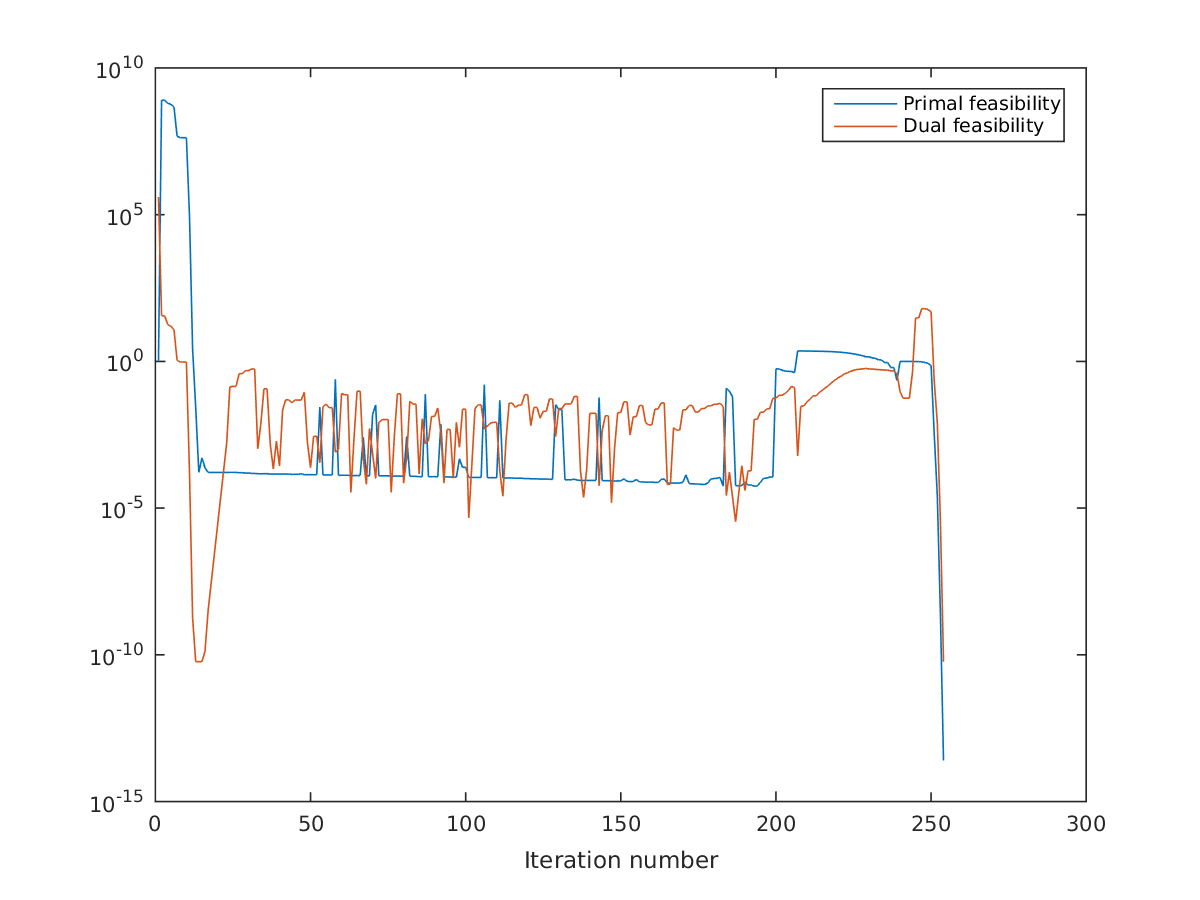##### On the importance of NLP scaling

During my master’s thesis at KULeuven on optimal control, one of the take-aways were that it’s important to scale your variables. It helps convergence if the variables are in the order of 0.01 to 100.##### Optimal control problems in a nutshell

Optimization. There’s a mathematical term that sounds familiar to the general public. Everyone can imagine engineers working hard to make your car run 1% more fuel-efficient, or to slightly increase profit margins for a chemical company.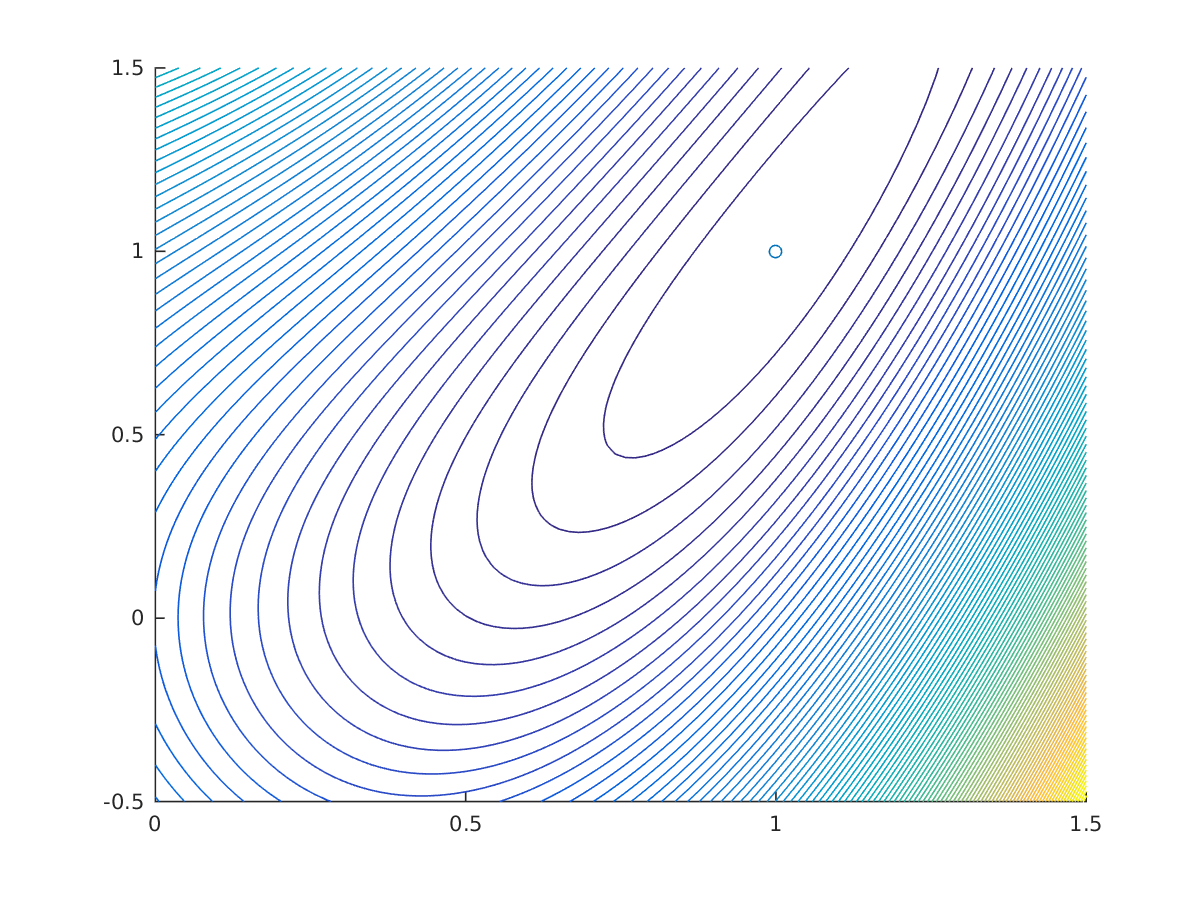##### Easy NLP modeling in CasADi with Opti

Release 3.3.0 of CasADi introduced a compact syntax for NLP modeling, using a set of helper classes, collectively known as ‘Opti stack’.

In this post, we briefly demonstrates this functionality.